# Performance Analysis of Five-Phase Induction Machine under Unbalanced Parameters

Performance Analysis of Five-Phase Induction Machine under Unbalanced Parameters
Laboratoire de Maitrise des Energies Renouvelables, Faculté de Technologie, Université de Bejaia 06000, Algérie

Ecole Supérieure des Sciences Appliquées d’Alger, Département Second Cycle, Alger 16000, Algérie

Corresponding Author Email:
lyes.khaldi@univ-bejaia.dz
Page:
521-526
|
DOI:
https://doi.org/10.18280/jesa.520512
22 June 2019
|
Accepted:
5 September 2019
|
Published:
13 November 2019
| Citation

OPEN ACCESS

Abstract:

The unbalance between parameters of multiphase induction motors is mainly resulted from the variation in the first-phase resistance of stator and rotor. This paper aims to examine the performance of five-phase induction machine with unbalanced parameters. First, a model was set up for the five-phase induction machine, which can efficiently identify the defects in natural frame of the machine. Then, five-phase induction machine were simulated in difference scenarios on Matlab/Simulink. The variations in stator and rotor resistance were measured, and their impacts on several performance indices were analyzed in details. The results show that the variations directly affect the torque ripples, Joule losses, and mechanical speed of the five-phase induction machine. The research findings indicate the possibility to eliminate this effect through fault-tolerant control.

Keywords:

performance analysis, five-phase induction machine, stator and rotor resistance variation, Joule losses, torque ripples, mechanical speed

1. Introduction

The multiphase induction machine has attracted considerable recent attention compared to the three phase induction machine, and it offers a lot of advantages such as: minimizing torque ripples, power segmentation and improved reliability [1-2].The multiphase machines are used for a high power application in the traction or propulsion, and during the last twenty years the research activity on multiphase drives has considered a large variety of multiphase motors. Although the five phase induction machine is probably the most studied for more time. A large of research has been directed towards the development of multiphase induction machines namely, the control [3-4], modeling [5-6], association machine-converter [7-8] and fault tolerance [9-10].

Multiphase induction motor parameters vary significantly with operating conditions [11-13]. The unbalance is usually due to an incipient fault, or a damage of the stator and rotor winding connections . The increase in the phase resistance can occur in any power connections of industrial electrical machines. In general terms, each electrical fault that occurs at the stator side makes the winding unsymmetrical because the phase impedances are not equal or because of distortions in the air gap flux density distribution [15-16]. The behavioral analysis is most frequently based on the development of a mathematical model that depicts the operation of the machine under fault. As discussed by Zarri et al.  the unbalance of stator winding is studied, based on the calculation of the space vectors of the stator currents in the available α-β planes, as shown by Apsley and Williamson  they have used a generalized harmonic analysis to provide accurate predictions of performance in multiphase induction machine. With the approach cited before, for simulating an unbalanced model it is necessary to add a new equation to the system. This method may influence the results, since the approach used to describe the new circuit introduce an estimated fault resistance . Or a model developed only in real coordinates (natural frame) as it will be presented in this proposed work, allow simulating all kinds of faults by only changing the parameters of the machine (resistors and inductances), without adding non-real components.

To solve these defects, this paper establishes a new modeling of the five phase induction machine in the natural frame (real frame) without adding new circuit or estimated resistance to simulate a fault, with the aim to examine the effect of stator and rotor resistance variations on the performance of five phase induction machine. The unbalance was created by the variation of the first phase resistance of stator and rotor. Each time the percentage of this resistance has been increased. It is indented to provide information about the impact of this unbalanced parameter on the torque ripples, Joule losses in the stator and rotor, and mechanical speed. The remainder of this paper is organized as follows: Section 2 a model of five phase induction machine has been presented, Section 3 describes the variation parametric of the machine, Section 4 presents a computer simulation to endorse the theoretical achievement given in Section 3 Finally some conclusions are made in Section 5.

2. Modeling of Five Phase Induction Machine Please

A schematic of the five-phase induction machine fed by five phase voltage sources is given in Figure 1. The stator and rotor winding of this machine are uniformly disturbed and displaced 2π/5.

The model we will adopt takes into account the following simplifying assumptions:

• The air gap is of uniform thickness;
• The notching effect is negligible;
• Magneto-motive force with sinusoidal spatial distribution;
• Symmetrical construction machine;
• Saturation of the magnetic circuit, hysteresis and eddy currents are negligible.

This approach consists of modeling the machine in the natural frame in a healthy diet. Then introduce a resistance in series with the phase in default. With this approach several faults can be simulated simultaneously.

The dynamic behavior of the five phase induction motor is described by the equations mentioned hereafter, where the voltage equations of five phase induction motor are expressed as follow:

$\left\{\begin{array}{l}{\left[v_{s}\right]=\left[r_{s}\right]\left[i_{s}\right]+\frac{d}{d t}\left[\psi_{s}\right]} \\ {\left[v_{r}\right]=\left[r_{r}\right]\left[i_{r}\right]+\frac{d}{d t}\left[\psi_{r}\right]}\end{array}\right.$   (1)

The flux equations of stator and rotor can be written as:

$\left[\begin{array}{l}{\left[\psi_{s}\right]} \\ {\left[\psi_{r}\right]}\end{array}\right]=\left[\begin{array}{cc}{\left[L_{s}\right]} & {\left[m_{s r}\right]} \\ {\left[m_{r s}\right]} & {\left[L_{r}\right]}\end{array}\right]\left[\begin{array}{l}{\left[i_{s}\right]} \\ {\left[i_{r}\right]}\end{array}\right]$  (2)

The mutual matrix inductance between stator and rotor is given as follows:

$\left[m_{s r}\right]_{5 x 5}=L_{m}\left[\begin{array}{ccccc}{\cos \left(\theta_{r}\right)} & {\cos \left(\theta_{r}-\frac{2 \pi}{5}\right)} & {\cos \left(\theta_{r}-\frac{4 \pi}{5}\right)} & {\cos \left(\theta_{r}-\frac{6 \pi}{5}\right)} & {\cos \left(\theta_{r}-\frac{8 \pi}{5}\right)} \\ {\cos \left(\theta_{r}-\frac{8 \pi}{5}\right)} & {\cos \left(\theta_{r}\right)} & {\cos \left(\theta_{r}-\frac{2 \pi}{5}\right)} & {\cos \left(\theta_{r}-\frac{4 \pi}{5}\right)} & {\cos \left(\theta_{r}-\frac{6 \pi}{5}\right)} \\ {\cos \left(\theta_{r}-\frac{6 \pi}{5}\right)} & {\cos \left(\theta_{r}-\frac{8 \pi}{5}\right)} & {\cos \left(\theta_{r}\right)} & {\cos \left(\theta_{r}-\frac{2 \pi}{5}\right)} & {\cos \left(\theta_{r}-\frac{4 \pi}{5}\right)} \\ {\cos \left(\theta_{r}-\frac{4 \pi}{5}\right)} & {\cos \left(\theta_{r}-\frac{6 \pi}{5}\right)} & {\cos \left(\theta_{r}-\frac{8 \pi}{5}\right)} & {\cos \left(\theta_{r}\right)} & {\cos \left(\theta_{r}-\frac{2 \pi}{5}\right)} \\ {\cos \left(\theta_{r}-\frac{2 \pi}{5}\right)} & {\cos \left(\theta_{r}-\frac{4 \pi}{5}\right)} & {\cos \left(\theta_{r}-\frac{6 \pi}{5}\right)} & {\cos \left(\theta_{r}-\frac{8 \pi}{5}\right)} & {\cos \left(\theta_{r}\right)} \end{array}\right]$   (3)

## 1.jpg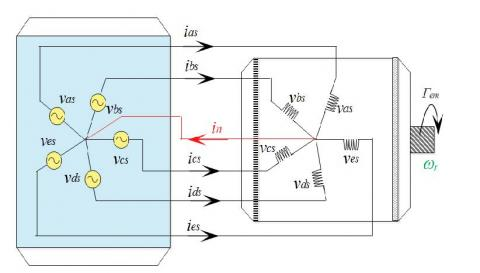Figure 1. Shame of five phase induction machine fed by five voltage sources

The stator inductance matrix is given as follows:

$\left[L_{s}\right]=L_{m}\left[\begin{array}{ccccc}{L_{s} / L_{m}} & {\cos \left(\frac{2 \pi}{5}\right)} & {\cos \left(\frac{4 \pi}{5}\right)} & {\cos \left(\frac{6 \pi}{5}\right)} & {\cos \left(\frac{8 \pi}{5}\right)} \\ {\cos \left(\frac{8 \pi}{5}\right)} & {L_{S} / L_{m}} & {\cos \left(\frac{2 \pi}{5}\right)} & {\cos \left(\frac{4 \pi}{5}\right)} & {\cos \left(\frac{6 \pi}{5}\right)} \\ {\cos \left(\frac{6 \pi}{5}\right)} & {\cos \left(\frac{8 \pi}{5}\right)} & {L_{S} / L_{m}} & {\cos \left(\frac{2 \pi}{5}\right)} & {\cos \left(\frac{4 \pi}{5}\right)} \\ {\cos \left(\frac{4 \pi}{5}\right)} & {\cos \left(\frac{6 \pi}{5}\right)} & {\cos \left(\frac{8 \pi}{5}\right)} & {L_{S} / L_{m}} & {\cos \left(\frac{2 \pi}{5}\right)} \\ {\cos \left(\frac{2 \pi}{5}\right)} & {\cos \left(\frac{4 \pi}{5}\right)} & {\cos \left(\frac{6 \pi}{5}\right)} & {\cos \left(\frac{8 \pi}{5}\right)} & {L_{s} / L_{m}}\end{array}\right]$   (4)

And that of the rotor is given by:

$\left[L_{r}\right]=L_{m}\left[\begin{array}{ccccc}{L_{r} / L} & {\cos \left(\frac{2 \pi}{5}\right)} & {\cos \left(\frac{4 \pi}{5}\right)} & {\cos \left(\frac{6 \pi}{5}\right)} & {\cos \left(\frac{8 \pi}{5}\right)} \\ {\cos \left(\frac{8 \pi}{5}\right)} & {L_{r} / L_{m}} & {\cos \left(\frac{2 \pi}{5}\right)} & {\cos \left(\frac{4 \pi}{5}\right)} & {\cos \left(\frac{6 \pi}{5}\right)} \\ {\cos \left(\frac{6 \pi}{5}\right)} & {\cos \left(\frac{8 \pi}{5}\right)} & {L_{r} / L_{m}} & {\cos \left(\frac{2 \pi}{5}\right)} & {\cos \left(\frac{4 \pi}{5}\right)} \\ {\cos \left(\frac{4 \pi}{5}\right)} & {\cos \left(\frac{6 \pi}{5}\right)} & {\cos \left(\frac{8 \pi}{5}\right)} & {L_{r} / L_{m}} & {\cos \left(\frac{2 \pi}{5}\right)} \\ {\cos \left(\frac{2 \pi}{5}\right)} & {\cos \left(\frac{4 \pi}{5}\right)} & {\cos \left(\frac{6 \pi}{5}\right)} & {\cos \left(\frac{8 \pi}{5}\right)} & {L_{r} / L_{m}}\end{array}\right]$   (5)

With:

$\left[m_{s r}\right]_{5 x 5}=\left[m_{s r}\right]_{5 x 5}^{t}$   (6)

And:

$\frac{d \theta_{r}}{d t}=P \Omega_{r}=\omega_{r}$   (7)

By replacing the Equation (2) into (1), and as the stator and rotor inductances matrices do not depend on time, and the mutual matrices depend of the electrical angle (θr), a variables change to develop the Equation (1) is established then the system equation can written as:

$\left\{\begin{array}{l}{\left[v_{s}\right]=\left[r_{s}\right]\left[i_{s}\right]+\left[L_{s}\right] \frac{d}{d t}\left[i_{s}\right]+\frac{d\left[i_{r}\right]}{d t}\left[m_{s r}\right]+\frac{\partial\left[m_{s r]}\right.}{\partial \theta_{r}} \frac{d \theta}{d t}\left[i_{r}\right]} \\ {=\left[r_{r}\right]\left[i_{r}\right]+\left[L_{r}\right] \frac{d}{d t}\left[i_{r}\right]+\frac{d\left[i_{s}\right]}{d t}\left[m_{r s}\right]+\frac{\partial\left[m_{r s}\right]}{\partial \theta_{r}} \frac{d \theta}{d t}\left[i_{s}\right]}\end{array}\right.$   (8)

After a few arrangements, finally we obtain a system of state which describes the behavior of electrical part of five phase induction machine such as:

\left\{\begin{aligned} \frac{d}{d t}\left[i_{s}\right] &=\left[L_{s}\right]^{-1}\left(\left[v_{s}\right]-\left[r_{s}\right]\left[i_{s}\right]-\frac{d\left[i_{r}\right]}{d t}\left[m_{s r}\right]-\frac{\partial\left[m_{s r}\right]}{\partial \theta} \frac{d \theta}{d t}\left[i_{r}\right]\right) \\ \frac{d}{d t}\left[i_{r}\right] &=\left[L_{r}\right]^{-1}\left(-\left[r_{r}\right]\left[i_{r}\right]-\frac{d\left[i_{s}\right]}{d t}\left[m_{r s}\right]-\frac{\partial\left[m_{r s}\right]}{\partial \theta} \frac{d \theta}{d t}\left[i_{s}\right]\right) \end{aligned}\right.  (9)

In order to design the complete model, it is necessary to integrate the mechanical equation characterizing the machine, or the mechanical system is expressed as follow:

$\Gamma_{e m}-\Gamma_{r}=J \frac{d \Omega_{r}}{d t}+f \Omega_{r}$  (10)

The electromagnetic torque is expressed as follow:

$\Gamma_{e m}=\frac{P}{2}\left(\left[i_{r}\right]^{t} \frac{\partial\left[m_{s r}\right]}{\partial \theta}\left[i_{s}\right]+\left[i_{r}\right]^{t} \frac{\partial\left[m_{r s}\right]}{\partial \theta}\left[i_{s}\right]\right)$  (11)

3. Variation Parametric of Five Phase Induction Machine

The performance of a five phase induction motor under balanced supply is simulated, where in this paper we based on variation parameters of stator and rotor resistances of five phase induction machine, or this unbalanced is created by varying the value of the resistance of the first phase stator and rotor of the machine, to analyze the impact of this variation on the torque ripples, Joule losses in the stator,and in the rotor and mechanical speed. In order to investigate the machine performance during variation parameter, a simulation model of five phase induction machine was introduced using MATLAB/Simulink, two simulation case was carried into effect where  each resistance is increased, 10%, 20%, 50%, 80% and 100% of stator and rotor resistances endependely.

4. Simulation Results and Interpretation

The Figure 2 present the schema of the simulation diagram, as well the Figure 3 and Figure 4 represent a simulation block of the stator and rotor currents equations respectively. The simulation block is developed under MATLAB/Simulink:

## 2.jpgFigure 2. Simulation diagram of the five phase induction machine in the natural frame

## 3.jpg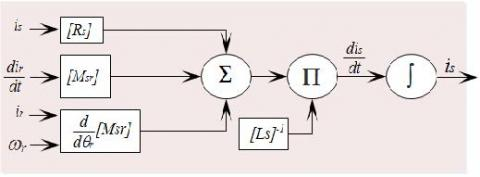Figure 3. Simulation block of the stator currents equation

## 4.jpg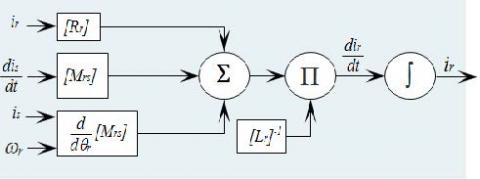Figure 4. Simulation block of the rotor currents equation

Table 1. Simulation results of variation stator resistance

 Per $R_{S}(\Omega)$ losses Joule stator (W) losses Joule rotor (W) Torque ripples (%) Speed (rd/s) 0% 4.850 165.400 100.090 0.000 150.830 10% 5.340 168.430 100.400 1.221 150.818 20% 5.850 171.490 100.720 2.432 150.805 50% 7.300 186.145 101.650 5.570 150.767 80% 8.730 186.440 102.580 8.255 150.733 100% 9.700 190.550 103.180 9.850 150.711

Table 2. Simulation results of variation rotor resistance

 Per $R_{r}(\Omega)$ losses Joule stator (W) losses Joule rotor (W) Torque ripples (%) Speed (rd/s) 0% 3.805 165.400 100.090 0.000 150.830 10% 4.180 165.890 101.760 3.070 150.810 20% 4.560 166.440 103.390 5.830 150.800 50% 5.700 168.110 107.460 12.470 150.770 80% 6.840 169.710 110.860 17.390 150.740 100% 7.610 170.620 112.820 20.041 150.720

The simulation results are mentioned in Table 1 and Table 2, if the machine state balanced it had no torque ripple, the speed has a value of 150.83 (rd/s) and the joule losses stator and rotor have values of 165.40 (W) and 100.09 (W) respectively. Then when the unbalanced was created, it brings a torque ripple, the increase Joule losses in the stator and the rotor and a decrease in the mechanical speed.

In conclusion, the machine degrades more and more every time the value of stator and/or rotor resistance was increased.

When the stator resistance was increased it affects the creation of an additional reverse and direct magnetic field in the machine of value frequency fs (where fs is the synchronism frequency), which will lead to torque and speed ripples with frequency of 2.fs .

As shown in Figure 5-8, and when the stator resistance was more increased the amplitude of the torque and speed ripples increases, or the current of the unbalance phase decrease.

## 5.png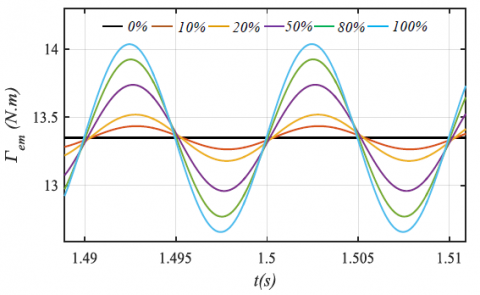Figure 5. Torque ripples under stator resistance variations

## 6.pngFigure 6. Mechanical speed under stator resistance variations

## 7.png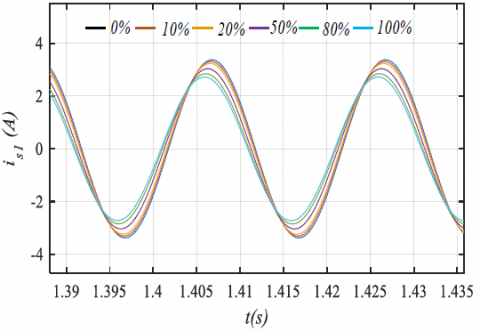Figure 7. Stator currents under stator resistance variations

## 8.png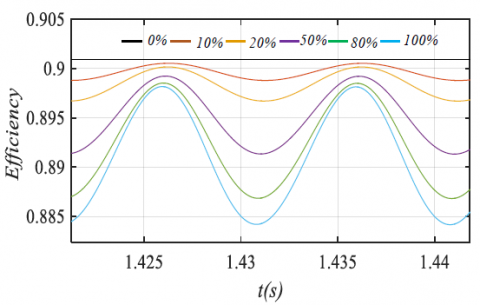Figure 8. Efficiency under stator resistance variations

## 9.png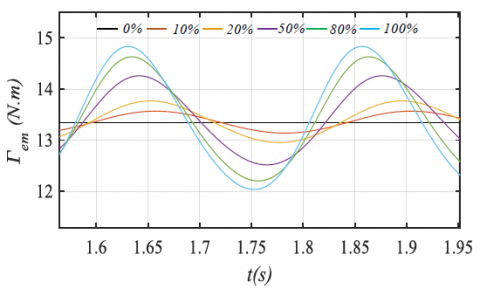Figure 9. Torque ripples under rotor resistance variations

## 10.png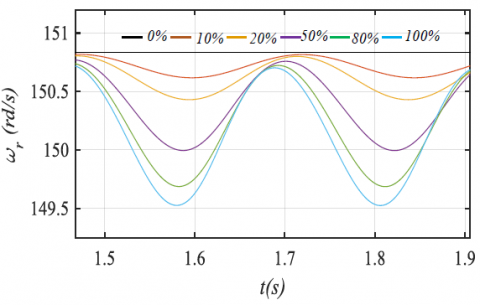Figure 10. Mechanical speed under rotor resistance variations

## 11.png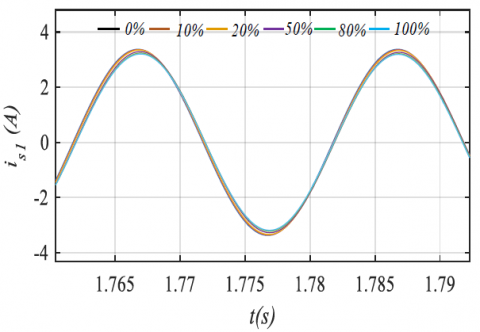Figure 11. Stator currents under rotor resistance variations

## 12.png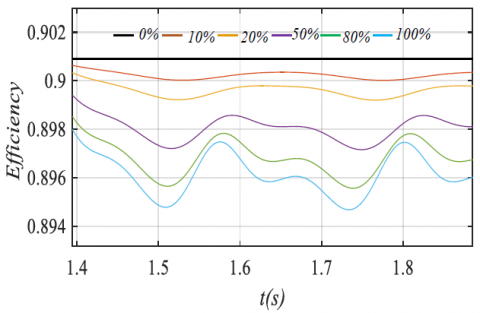Figure 12. Efficiency under rotor resistance variations

The Figure 9-12 shows the torque ripples, speed and current of the unbalance phase when the rotor resistance was increased, when this resistance was increased its affect to the rotor currents that generate an inverse magnetic field of frequency–g.fs(where g is slip), therefore currents at the frequencies (1-2g)fs are induced in the stator circuit, accompanied by a torque and speed ripples of 2.g.fs frequency . The amplitude of the torque and speed ripples increases with a phase shift between these ripples when resistance was more increased, this since each time the resistance was increased the slip increased as shown in Figure 15 which creates a phase shift.

## 13.jpg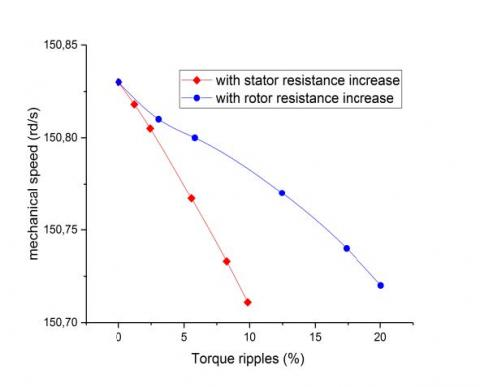Figure 13. Mechanical speed according to torque ripples

## 14.jpgFigure 14. Torque ripples with stator and rotor resistance increase

## 15.jpg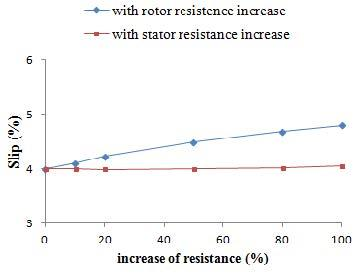Figure 15. Slip under stator and rotor resistance variations

The torque ripples are greater during variation of rotor resistances then stator resistance according to Figure 14. As shown in Figure 13 the mechanical speed decrease more when the torque ripples increase, knowing that this is more important when the rotor resistance was increased because the torque ripples are grater in this case. The joule losses in stator increase when the stator resistance was increased that was noticed according to Figure 17, because the resistance of one phase was increased and also the currents of other phase increased, and followed by very small joule losses in the rotor that we can neglect, because the current was underwent a lightest increase. As shown in the Figure 16 the joule losses in the rotor increase when the rotor resistance was increased accompanied by small increase in stator Joule losses, because as mentioned before when the slip increase which leads to increase of the stator current causes an increase in the intensity of the current in the rotor.

## 16.jpgFigure 16. Losses joule in rotor under stator and rotor resistance variations

## 17.jpg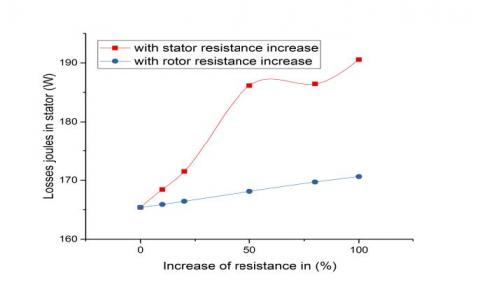Figure 17. Losses joule in stator under stator and rotor resistance variations

5. Conclusions

This paper has presented a performance analysis of five phase induction machine under unbalanced parameters. Simulation result under MATLAB/Simulink for both healthy and under unbalanced parameters of five phase Induction machine have been presented to justify the validity of the proposed approach.

The simulation results show the effect of stator and rotor resistance variation while focusing on their genesis, where it has always as result a ripple torque increase, an increase Joule loss in the stator and rotor, a decrease in mechanical speed. Or in the case of a variation of rotor resistance the effects are more intense since the ripples have a phase shift because there is a variation of slip.

Nomenclature
 $f$ Viscous friction $J$ Inertia $i_{s}$ $i_{r}$ $L_{m}$ $L_{s}$ $L_{r}$ $P$ $R_{r}$ $R_{s}$ $v_{r}$ $v_{s}$ Stator currents Rotor currents Magnetizing inductance Per phase stator leakage inductance Per phase rotor leakage inductance Number of pole pairs Rotor resistances Stator resistances Rotor voltage Stator voltage Greek symbols $\psi_{r}$ Rotor flux $\psi_{S}$ Stator flux $\theta_{r}$ Electrical angle $\Gamma_{e m}$ Electromagnetic torque $\omega_{r}$ Rotor electrical speed $\Omega_{r}$ Rotor mechanical speed
References

 Levi, E., Bojoi, R., Profumo, F., Toliyat, H.A., Williamson, S. (2007). Multiphase induction motor drives – a technology status review. IET Electric Power Applications, 1(4): 489-516. https://doi.org/10.1049/iet-epa:20060342

 Amimeur, H., Aouzellag, D., Abdessemed, R., Ghedamsi, K. (2012). Sliding mode control of a dual star induction generator for wind energy conversion systems. Electrical Power and Energy Systems, 42: 60-70. https://doi.org/10.1016/j.ijepes.2012.03.024

 Rahali, H., Zeghlache, S., Benalia, L., Layadi, N. (2018). Sliding mode control based on back stepping approach for a double star induction motor (DSIM). Advanced in Modeling and Analysis C, 73(04): 150-157. https://doi.org/10.18280/ama_c.730404

 Hellali, L., Belmahdi, S., Loutfi, B., Hassen, R. (2018). Direct torque control of doubly star induction machine fed by voltage source inverter using type-2 fuzzy logic speed controller. Advanced in Modeling and Analysis C,73(04): 202-207. https://doi.org/10.18280/ama_c.7304 10

 Iffouzar, K., Benkhoris, M.F., Ghedamsi, K., Aouzellag, D. (2016). Behaviour analysis of dual stars induction motor supplied by PWM multilevel inverters. Rev. Roum. Sci. Techn. – Électrotechn. Et Énerg., 61(2): 137-141.

 Rajinder, E.A., Sreejeth, M., Singh, M. (2016). Sensitivity analysis of induction motor performance variables. 1st IEEE International Conference on Power Electronics, Intelligent Control and Energy Systems (ICPEICES-2016).

 Iffouzar, K., Taraft, S., Aouzellag, H., Ghedamsi, K., Aouzellag, D. (2015). Behavior of six phase induction motor fed by multilevel inverter. International conference of Electrical Engineering, ICEE 15, Algeria.

 Levi, E. (2016). Advances in converter control and innovative exploitation of additional degrees of freedom for multiphase machines. IEEE Trans. Ind. Electron., 63(1): 433-448. https://doi.org/10.1109/TIE.2015.24349 99

 Mahmoud, E.A., Abdel-Khalik, A.S., Soliman, H.F. (2016). Improved fault tolerant for a five phase induction machine under open gate transistor faults. Alexandria Engineering Journal, 55(3): 2609-2620. https://doi.org/10.1016/j.aej.2016.04.040

 Rangari, R.C., Suryawanshi, H.M. (2018). New fault control strategy of five phase induction motor with four-phase and three-phase modes operation. Jurnal of Eectronics, 7(9): 159. https://doi.org/10.3390/electronics7090159

 Mengoni, M., Zarri, L., Tani, A., Gritli, Y., Serra, G., Filippetti, F. (2012). Behaviour of mltiphase iduction mchines with ubalanced sator rsistances. 15th International Power Electronics and Motion Control Conference, Novi Sad, Serbia. https://doi.org/10.1109/EPEPEMC.2012.6397475

 Abdel-Khalik, A.S., Morsy, A., Ahmed, S., Massoud, A. (2014). Effect of stator winding connection on performance of five phase induction machine. IEEE Trans on Industrial Electronics, 61(1): 3-19 https://doi.org/10.1109/TIE.2013.2242417

 Abdel-Khalik, A.S., Ahmed S., Elserougi, A., Massoud, A. (2015). Effect of stator winding connection of five phase induction machine on torque ripples under open line condition. IEEE/ASME Trans. Mechatronic, 20(2): 580-593. https://doi.org/10.1109/TMECH.2014.2303254

 Abdel-Khalik, A.S., Massoud, A., Ahmed, S. (2016). A senior project-based multiphase motor drive system development. IEEE, Trans on Education, 59(4): 307-318. https://doi.org/10.1109/TE.2016.2555288

 Baneira, F., Doval-Gando, J., Yepes, A., Lopez, O., Perez-Estevez, D. (2017). Control strategy for multiphase drives with minimum losses in the full torque operation range under single open-phase fault. IEEE Trans on Power Electronic, 32(8): 6275-6285. https://doi.org/10.1109/TPEL.2016.2620426

 Duran, M.J., Barrero, F. (2017). Recent advances in the design, modelling and control of multiphase machines. IEEE Trans on Industrial Electronics, (63):1: 449-458. https://doi.org/10.1109/TIE.2015.2448211

 Zarri, L., Mengoni, M., Gritli, Y., Tani, A., Filippetti, F., Serra, G., Casadei, D. (2011). Behavior of multiphase induction machines with unbalanced stator windings. 8th IEEE Symposium on Diagnostics for Electrical Machines, Power Electronics & Drives, Sept. Bologna, Italy. https://doi.org/10.1109/DEMPED.2011.6063606

 Apsley, J.M., Williamson, S. (2005). Analysis of multi-phase induction machines with winding faults. IEEE International Conference on Electric Machines and Drives, San Antonio, TX, USA. https://doi.org/10.1109/IEMDC.2005.195731

 Vukosavic, S.N., Jones, M., Levi, E., Varga, J. (2005). Rotor flux oriented control of a symmetrical six-phase induction machine. Electric Power Systems Research, 75(2-3): 142-152. https://doi.org/10.1016/j.epsr.2005.02.006

 Siddique, A., Yadava, G.S., Singh, B. (2005). A review of stator fault monitoring technique of induction motors. IEEE Trans on Energy Conversion, 20(1): 106-114. https://doi.org/10.1109/TEC.2004.837304

 Sahraouian, M., Zouzou, S., Menacer, A. (2004). Fault diagnosis in three phase squirrel cage asynchronous motors part II: Methods for detecting bulbar breaks in three phase squirrel cage asynchronous motors. Mail of Knowledge, 05(1): 57-61.